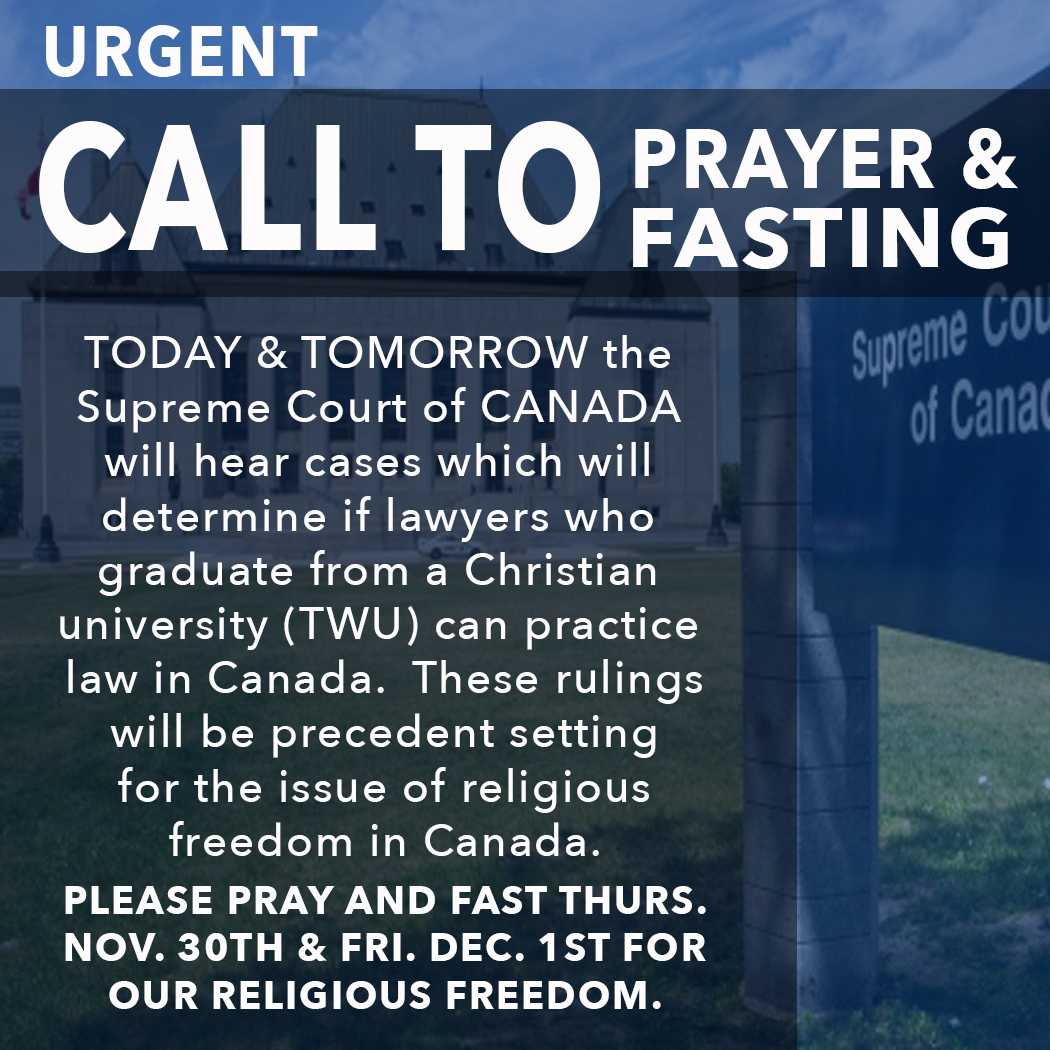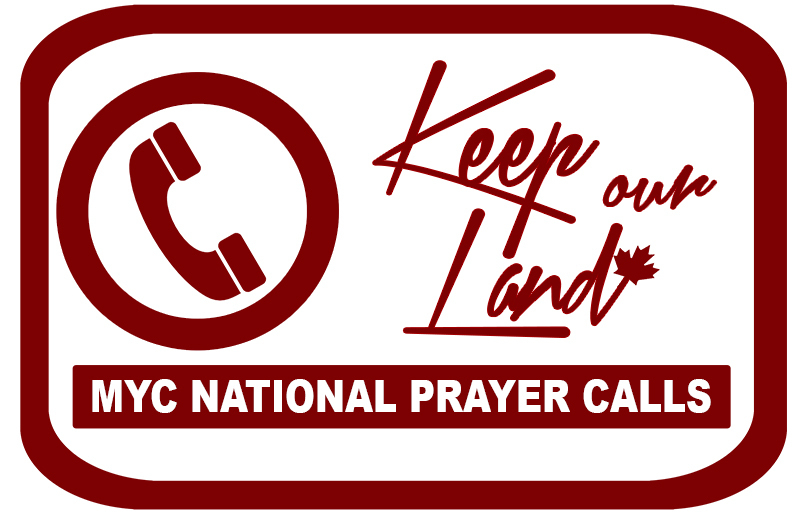Like   Tweet   Pin   +1   in
 /* styles */ November 30th, 2017

Today is a critical day for religious freedom in Canada as the first of two Trinity Western University law school court cases goes before the Supreme Court of Canada.

MY Canada is calling on our members to pray and if possible to fast for the lawyers, judges, TWU leadership, and religious freedom in Canada throughout the day today as well as tomorrow, when the second TWU case is heard.

## National Leaders & Organizations Join the Call to Prayer:

Click below to watch our National Director, Faytene Grasseschi, explain the significance of the TWU law school situation and potential implications for religious freedom in Canada.

 table div table+table+table+table div table td,table.module-3{width:100%;padding:0}table div table+table+table+table div table{width:100%;float:none;margin-left:auto;margin-right:auto;padding:0}table div table+table+table+table div table a{border:0 none;text-decoration:none}table div table+table+table+table div table img{width:100%!important;border:0 none;text-decoration:none}/* styles */
 /* styles */ Pastors across the nation, including Pastor Matt Tapley of Lakemount Worship Centre in Grimbsy, ON, have sent out similar calls to prayer urging their members to pray for the TWU cases. Please click below to watch now.
 table div table+table+table+table+table+table div table td,table.module-5{width:100%;padding:0}table div table+table+table+table+table+table div table{width:100%;float:none;margin-left:auto;margin-right:auto;padding:0}table div table+table+table+table+table+table div table a{border:0 none;text-decoration:none}table div table+table+table+table+table+table div table img{width:100%!important;border:0 none;text-decoration:none}/* styles */
 /* styles */ ARPA Canada's Director of Law and Policy, Andre Schutten, is a lawyer who has been granted intervenor status in the two TWU appeals to be heard this week. In the broadcast below (beginning at 7:20), Andre gives a backgrounder and some critical information on the battle for religious freedom surrounding TWU's proposed law school. Please click the viewer below to listen to this important interview.
 table.module-7{width:99.81%;padding:0}table div table+table+table+table+table+table+table+table div table{width:99.81%;float:none;margin-left:auto;margin-right:auto;padding:0}table div table+table+table+table+table+table+table+table div table a{border:0 none;text-decoration:none}table div table+table+table+table+table+table+table+table div table img{width:100%!important;border:0 none;text-decoration:none}table div table+table+table+table+table+table+table+table div table td{width:100%;padding:0}/* styles */

▪ For wisdom and insight for the judges, lawyers and interveners on both sides in the TWU case.
▪ That the Supreme Court of Canada will rule that there is no valid reason that TWU law schools should not be accredited.
▪ That the precedent that is set will be one for religious freedom in Canada.
▪ For the churches of Canada; that we would be compassionate and godly citizens representing Jesus Christ well, even as our country changes around us.
▪ For the provincial and federal governments of Canada; that they would know God’s wisdom and govern with insight and compassion for all the citizens of Canada.
 ▪ For wisdom and insight for the judges, lawyers and interveners on both sides in the TWU case.
 ▪ That the Supreme Court of Canada will rule that there is no valid reason that TWU law schools should not be accredited.
 ▪ That the precedent that is set will be one for religious freedom in Canada.
 ▪ For the churches of Canada; that we would be compassionate and godly citizens representing Jesus Christ well, even as our country changes around us.
 ▪ For the provincial and federal governments of Canada; that they would know God’s wisdom and govern with insight and compassion for all the citizens of Canada.

## Shareable Social Media Graphic:table div table+table+table+table+table+table+table+table+table+table+table div table{width:100%;padding:0}table div table+table+table+table+table+table+table+table+table+table+table div table img{width:96.23%;padding:0;float:none}table div table+table+table+table+table+table+table+table+table+table+table div table td{width:100%;padding:0 1.88% 18px}/* styles */# Thank you for standing with us in prayer for religious freedom in Canada during this defining moment.

 table.module-12{width:98.49%;padding:0}table div table+table+table+table+table+table+table+table+table+table+table+table+table div table{width:98.49%;float:none;margin-left:auto;margin-right:auto;padding:0}table div table+table+table+table+table+table+table+table+table+table+table+table+table div table a{border:0 none;text-decoration:none}table div table+table+table+table+table+table+table+table+table+table+table+table+table div table img{width:100%!important;border:0 none;text-decoration:none}table div table+table+table+table+table+table+table+table+table+table+table+table+table div table td{width:100%;padding:0}/* styles */
 table div table+table+table+table+table+table+table+table+table+table+table+table+table+table div table{width:100%;padding:0}table div table+table+table+table+table+table+table+table+table+table+table+table+table+table div table img{width:96.23%;padding:0;float:none}table div table+table+table+table+table+table+table+table+table+table+table+table+table+table div table td{width:100%;padding:0 1.88% 18px}/* styles */## ANNOUNCEMENTS:

 table div table+table+table+table+table+table+table+table+table+table+table+table+table+table+table+table div table{width:100%;padding:0}table div table+table+table+table+table+table+table+table+table+table+table+table+table+table+table+table div table img{width:96.23%;padding:0;float:none}table div table+table+table+table+table+table+table+table+table+table+table+table+table+table+table+table div table td{width:100%;padding:0 1.88% 18px}/* styles */## Interested in Running for Election?

We can help! MY Canada is currently conducting a series of trainings and webinars to help godly candidates get started in their campaigns for office. Please e-mail admin@4mycanada.ca if you are interested in learning more.

 table div table+table+table+table+table+table+table+table+table+table+table+table+table+table+table+table+table+table div table{width:100%;padding:0}table div table+table+table+table+table+table+table+table+table+table+table+table+table+table+table+table+table+table div table img{width:96.23%;padding:0;float:none}table div table+table+table+table+table+table+table+table+table+table+table+table+table+table+table+table+table+table div table td{width:100%;padding:0 1.88% 18px}/* styles */## Volunteers Needed:

 table div table+table+table+table+table+table+table+table+table+table+table+table+table+table+table+table+table+table+table+table div table{width:100%;padding:0}table div table+table+table+table+table+table+table+table+table+table+table+table+table+table+table+table+table+table+table+table div table img{width:96.23%;padding:0;float:none}table div table+table+table+table+table+table+table+table+table+table+table+table+table+table+table+table+table+table+table+table div table td{width:100%;padding:0 1.88% 18px}/* styles */table.module-20{width:99.81%;padding:0}table div table+table+table+table+table+table+table+table+table+table+table+table+table+table+table+table+table+table+table+table+table div table{width:99.81%;float:none;margin-left:auto;margin-right:auto;padding:0}table div table+table+table+table+table+table+table+table+table+table+table+table+table+table+table+table+table+table+table+table+table div table a{border:0 none;text-decoration:none}table div table+table+table+table+table+table+table+table+table+table+table+table+table+table+table+table+table+table+table+table+table div table img{width:100%!important;border:0 none;text-decoration:none}table div table+table+table+table+table+table+table+table+table+table+table+table+table+table+table+table+table+table+table+table+table div table td{width:100%;padding:0}/* styles */
 /* styles */ The new Justice Wall website is now live and allows for many thousands of individuals to sign up to pray for the ending of abortion and human trafficking and for the persecuted Church around the world. Will you help us build a 24-7 virtual prayer wall for these issues? Click here or visit www.JusticeWall.com to sign up for your 15-minute prayer slot now.
 table div table+table+table+table+table+table+table+table+table+table+table+table+table+table+table+table+table+table+table+table+table+table+table div table{width:100%;padding:0}table div table+table+table+table+table+table+table+table+table+table+table+table+table+table+table+table+table+table+table+table+table+table+table div table img{width:96.23%;padding:0;float:none}table div table+table+table+table+table+table+table+table+table+table+table+table+table+table+table+table+table+table+table+table+table+table+table div table td{width:100%;padding:0 1.88% 18px}/* styles */table div table+table+table+table+table+table+table+table+table+table+table+table+table+table+table+table+table+table+table+table+table+table+table+table+table div table{width:100%;padding:0}table div table+table+table+table+table+table+table+table+table+table+table+table+table+table+table+table+table+table+table+table+table+table+table+table+table div table img{width:96.23%;padding:0;float:none}table div table+table+table+table+table+table+table+table+table+table+table+table+table+table+table+table+table+table+table+table+table+table+table+table+table div table td{width:100%;padding:0 1.88% 18px}/* styles */MY Canada is hosting national prayer conference calls every second Tuesday at noon EST.

The next call is December 5th, 2017.

 table div table+table+table+table+table+table+table+table+table+table+table+table+table+table+table+table+table+table+table+table+table+table+table+table+table+table+table div table{width:100%;padding:0}table div table+table+table+table+table+table+table+table+table+table+table+table+table+table+table+table+table+table+table+table+table+table+table+table+table+table+table div table img{width:96.23%;padding:0;float:none}table div table+table+table+table+table+table+table+table+table+table+table+table+table+table+table+table+table+table+table+table+table+table+table+table+table+table+table div table td{width:100%;padding:0 1.88% 18px}/* styles */table div table+table+table+table+table+table+table+table+table+table+table+table+table+table+table+table+table+table+table+table+table+table+table+table+table+table+table+table+table div table{width:100%;padding:0}table div table+table+table+table+table+table+table+table+table+table+table+table+table+table+table+table+table+table+table+table+table+table+table+table+table+table+table+table+table div table img{width:96.23%;padding:0;float:none}table div table+table+table+table+table+table+table+table+table+table+table+table+table+table+table+table+table+table+table+table+table+table+table+table+table+table+table+table+table div table td{width:100%;padding:0 1.88% 18px}/* styles */